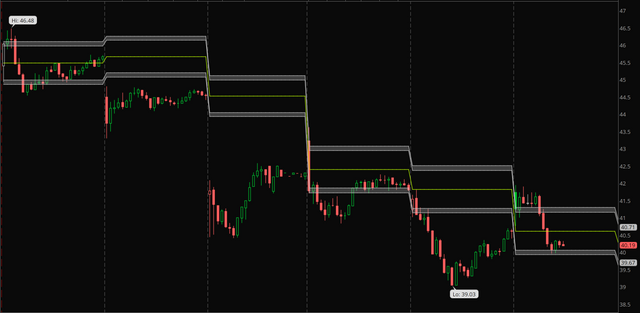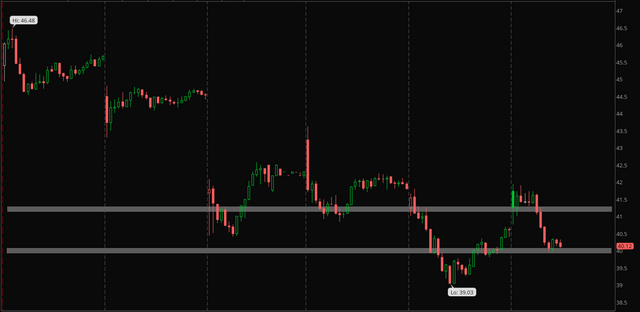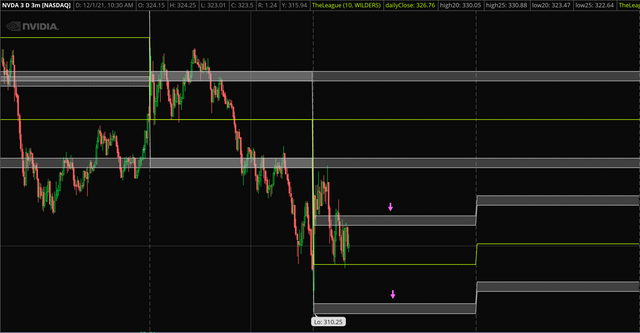# Plot cloud across weeks/months instead of just day

#### roughsleeper

##### New member
Hey all,

I made a quick study that shows a range I'm looking at for each day, and it's working out nicely per day, but I'd like to plot these clouds/lines in a different way.

I'm trying to plot only yesterday's levels across the entire chart (including today) – back weeks or months – and hide every other level previous to yesterday's levels.

Here's a visual of where I'm at:and here's what I'm trying to make happen (with lines / clouds etc. same as above) – take yesterday's levels and apply them all the way back. I can't figure out how to do it.And the code:

Code:
``````input length = 10;
input averageType = AverageType.WILDERS;

def ATR20 = MovingAverage(averageType, TrueRange(high(period=”DAY”), close(period=”DAY”), low(period=”DAY”)), length)/5;
def ATR25 = MovingAverage(averageType, TrueRange(high(period=”DAY”), close(period=”DAY”), low(period=”DAY”)), length)/4;

plot dailyClose = close(period=”DAY”);

plot high20 = dailyClose + ATR20;
plot high25 = dailyClose + ATR25;

plot low20 = dailyClose - ATR20;
plot low25 = dailyClose - ATR25;

dailyClose.SetDefaultColor(color.LIME);
high20.SetDefaultColor(color.LIGHT_GRAY);
low20.SetDefaultColor(color.LIGHT_GRAY);
high25.SetDefaultColor(color.LIGHT_GRAY);
low25.SetDefaultColor(color.LIGHT_GRAY);``````

any help is appreciated! thanks!

•andreythedon
Solution
Hey all,

I made a quick study that shows a range I'm looking at for each day, and it's working out nicely per day, but I'd like to plot these clouds/lines in a different way.

I'm trying to plot only yesterday's levels across the entire chart (including today) – back weeks or months – and hide every other level previous to yesterday's levels.

Here's a visual of where I'm at:and here's what I'm trying to make happen (with lines / clouds etc. same as above) – take yesterday's levels and apply them all the way back. I can't figure out how to do it.And the code:

Code:
``````input length = 10;
input averageType = AverageType.WILDERS;

def ATR20 = MovingAverage(averageType, TrueRange(high(period=”DAY”)...``````
Hey all,

I made a quick study that shows a range I'm looking at for each day, and it's working out nicely per day, but I'd like to plot these clouds/lines in a different way.

I'm trying to plot only yesterday's levels across the entire chart (including today) – back weeks or months – and hide every other level previous to yesterday's levels.

Here's a visual of where I'm at:and here's what I'm trying to make happen (with lines / clouds etc. same as above) – take yesterday's levels and apply them all the way back. I can't figure out how to do it.And the code:

Code:
``````input length = 10;
input averageType = AverageType.WILDERS;

def ATR20 = MovingAverage(averageType, TrueRange(high(period=”DAY”), close(period=”DAY”), low(period=”DAY”)), length)/5;
def ATR25 = MovingAverage(averageType, TrueRange(high(period=”DAY”), close(period=”DAY”), low(period=”DAY”)), length)/4;

plot dailyClose = close(period=”DAY”);

plot high20 = dailyClose + ATR20;
plot high25 = dailyClose + ATR25;

plot low20 = dailyClose - ATR20;
plot low25 = dailyClose - ATR25;

dailyClose.SetDefaultColor(color.LIME);
high20.SetDefaultColor(color.LIGHT_GRAY);
low20.SetDefaultColor(color.LIGHT_GRAY);
high25.SetDefaultColor(color.LIGHT_GRAY);
low25.SetDefaultColor(color.LIGHT_GRAY);``````

any help is appreciated! thanks!
here is one way

Ruby:
``````def na = double.nan;
def bn = barnumber();
# -----------------------------
# define yesterday
def Yesterday = if GetDay() == (GetLastDay() - 1) then 1 else 0;
input include_after_hour_data = no;
def rth = if secondsFromTime(0930) >= 0 and secondsTillTime(1600) > 0 then 1 else 0;
def getdata = if include_after_hour_data then 1 else rth;
def prevday = (yesterday == 1 and getdata == 1);
# -------------------------------
input length = 10;
input averageType = AverageType.WILDERS;
def ATR20 = MovingAverage(averageType, TrueRange(high(period=”DAY”), close(period=”DAY”), low(period=”DAY”)), length)/5;
def ATR25 = MovingAverage(averageType, TrueRange(high(period=”DAY”), close(period=”DAY”), low(period=”DAY”)), length)/4;
def dailyClose = if prevday then close(period = "DAY") else na;
def high20 =  if prevday then (dailyClose + ATR20) else na;
def high25 =  if prevday then (dailyClose + ATR25) else na;
def low20 =  if prevday then (dailyClose - ATR20) else na;
def low25 =  if prevday then (dailyClose - ATR25) else na;

plot dcls = highestall(dailyclose);
plot h20 = highestall(high20);
plot h25 = highestall(high25);
plot l20 = highestall(low20);
plot l25 = highestall(low25);

dcls.SetDefaultColor(color.LIME);
h20.SetDefaultColor(color.LIGHT_GRAY);
l20.SetDefaultColor(color.LIGHT_GRAY);
h25.SetDefaultColor(color.LIGHT_GRAY);
l25.SetDefaultColor(color.LIGHT_GRAY);

#-------------------------------
input show_line_above_day = no;
plot w = if (show_line_above_day  and prevday) then ( 1.000 * high(period = "DAY") ) else na;
w.SetDefaultColor(Color.light_gray);

# ---------------------------
input test_show_day_dots = no;
plot z = if (test_show_day_dots and prevday) then (low*0.99) else na;
z.SetPaintingStrategy(PaintingStrategy.points);
z.SetDefaultColor(Color.cyan);
z.setlineweight(3);
#``````

borrowed from this code

•roughsleeper
here is one way

Ruby:
``````def na = double.nan;
def bn = barnumber();
# -----------------------------
# define yesterday
def Yesterday = if GetDay() == (GetLastDay() - 1) then 1 else 0;
input include_after_hour_data = no;
def rth = if secondsFromTime(0930) >= 0 and secondsTillTime(1600) > 0 then 1 else 0;
def getdata = if include_after_hour_data then 1 else rth;
def prevday = (yesterday == 1 and getdata == 1);
# -------------------------------
input length = 10;
input averageType = AverageType.WILDERS;
def ATR20 = MovingAverage(averageType, TrueRange(high(period=”DAY”), close(period=”DAY”), low(period=”DAY”)), length)/5;
def ATR25 = MovingAverage(averageType, TrueRange(high(period=”DAY”), close(period=”DAY”), low(period=”DAY”)), length)/4;
def dailyClose = if prevday then close(period = "DAY") else na;
def high20 =  if prevday then (dailyClose + ATR20) else na;
def high25 =  if prevday then (dailyClose + ATR25) else na;
def low20 =  if prevday then (dailyClose - ATR20) else na;
def low25 =  if prevday then (dailyClose - ATR25) else na;

plot dcls = highestall(dailyclose);
plot h20 = highestall(high20);
plot h25 = highestall(high25);
plot l20 = highestall(low20);
plot l25 = highestall(low25);

dcls.SetDefaultColor(color.LIME);
h20.SetDefaultColor(color.LIGHT_GRAY);
l20.SetDefaultColor(color.LIGHT_GRAY);
h25.SetDefaultColor(color.LIGHT_GRAY);
l25.SetDefaultColor(color.LIGHT_GRAY);

#-------------------------------
input show_line_above_day = no;
plot w = if (show_line_above_day  and prevday) then ( 1.000 * high(period = "DAY") ) else na;
w.SetDefaultColor(Color.light_gray);

# ---------------------------
input test_show_day_dots = no;
plot z = if (test_show_day_dots and prevday) then (low*0.99) else na;
z.SetPaintingStrategy(PaintingStrategy.points);
z.SetDefaultColor(Color.cyan);
z.setlineweight(3);
#``````

borrowed from this code

Oh whoa thank you so much halcyonguy! I really appreciate that. This is looking so good.

I might've misspoke in my post, I'm looking to have the current day's range plotted for the next trading day. So basically the idea is, take today's close, figure out 20/25% ATR from (x) days back, and create bands on tomorrow's day period that are 20-25% ATR above and below current day's close.

Here are both overlaid – I'm trying to get the levels from the pink arrow area plotted across (+ also check the areas that are currently being plotted for tomorrow):thanks again

**Err I think I may have figured it out by changing all of your 's to 's – does that seem right?

Last edited by a moderator:

### Not the exact question you're looking for?

87k+ Posts
352 Online## The Market Trading Game Changer

Join 2,500+ subscribers inside the useThinkScript VIP Membership Club
• Exclusive indicators
• Proven strategies & setups
• Private Discord community
• Exclusive members-only content
• 1 full year of unlimited support

What is useThinkScript?

useThinkScript is the #1 community of stock market investors using indicators and other tools to power their trading strategies. Traders of all skill levels use our forums to learn about scripting and indicators, help each other, and discover new ways to gain an edge in the markets.

How do I get started?

We get it. Our forum can be intimidating, if not overwhelming. With thousands of topics, tens of thousands of posts, our community has created an incredibly deep knowledge base for stock traders. No one can ever exhaust every resource provided on our site.

If you are new, or just looking for guidance, here are some helpful links to get you started.

What are the benefits of VIP Membership?